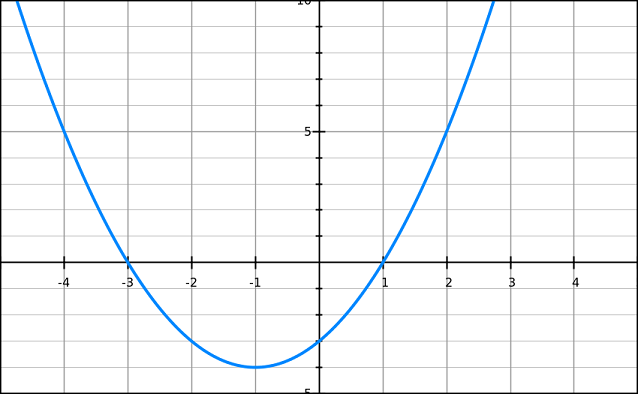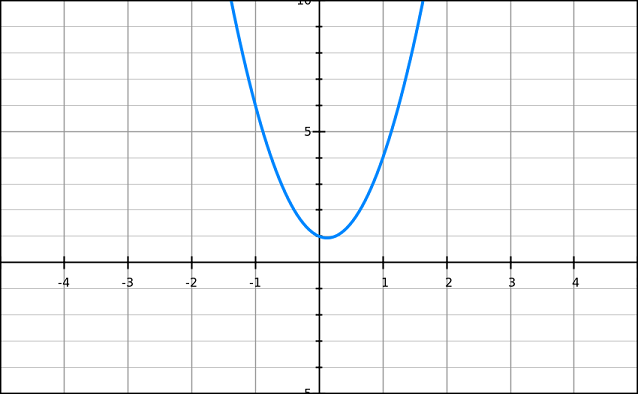### What is a quadratic equation?

The quadratic formula is used to solve a very specific type of equation, called a quadratic equation. These equations are usually written in the following form, where A, B, and C are constants and x represents an unknown.

$$Ax^2 + Bx + C = 0$$

Quadratic equations are second-order polynomials (the highest exponent is two) with a single unknown (x). In simple terms, that means that there's an $$x^2$$ term, but no $$x^3$$ or $$x^4$$ terms, etc. They may or may not have all three terms present ($$Bx$$ or $$C$$ may be absent). There are several options to solve a quadratic equation for x, including factoring or completing the square. In this lesson we will use the aptly-named quadratic formula, which takes the three coefficients (A, B, and C) and provides up to two solutions, if they exist.

The first step to using the quadratic formula will be identifying the three coefficients, A, B, and C. In the equation above they are conveniently all together on one side of a simplified equation. This will be the easiest way of identifying their values. For example, this is a simple quadratic equation:

$$2x^2 - 8x + 7 = 0$$

Notice that I've replaced $$A$$ with 2, $$B$$ with -8, and $$C$$ with 7. Finding those three value gets us halfway to the answer. Sometimes, however the three coefficients may not all be present, or there may be some simplification required. For example, what if we were given the following equation and asked to solve?

$$2x^2+x+7=2x-6$$

We would first need to re-write that equation into a simplified form with a single coefficient in front of each term. In this case, that means subtracting 2x from each side and adding 6 to each side:

$$2x^2-x+13=0$$

Now we have the equation in a standard form where we can identify that A=2, B=-1, and C=13.

To practice identifying the coefficients, the following are examples of quadratic equations. Can you identify the values of A, B, and C in each?

$$2x^2 + 3x + 5 = 0$$ $$x^2 + 3x + 4 = 0$$ $$x^2 - 4x + 4 = 0$$ $$2x^2 - 10 = 0$$ $$x^2 + 5x = -8$$

### Solving the equation for x

How would you solve that equation for x? We have simplified it to a standard form, but there's no obvious way to manipulate that equation into an x= form. This is where the quadratic formula comes in handy!

The quadratic formula is a tool we can use to solve quadratic equations for any possible solutions. We'll eventually discover that there may be more than one unique solution, in fact, or none at all. Here it is:

$$x = \frac{-b \pm \sqrt{b^2-4ac}}{2a}$$

It looks really complicated, I know. Once you use it a lot you'll learn the formula by heart, but until then keep practicing. Actually using it isn't that hard -- just plug in your numbers step-by-step. We learned already to find the three coefficients A, B, and C, and I said we were halfway to a solution. Now we just insert the values for A,B, and C and we'll be left with two possible solutions for x, if they exist. Why two? Notice that there is a plus/minus sign in the formula. That means we need to account for both possibilities when solving.

Watch how I solve a quadratic equation below, and try to follow along.

### Example:

Solve this equation for x: $$x^2 - 4x + 4 = 0$$

### Solution:

In order to solve with our formula, we must identify the three coefficients (A,B,C) in order to begin solving. Remember that A is the coefficient in front of the $$x^2$$ term, B is the coefficient in front of $$x$$, and C is the constant at the end.

Therefore, for this equation, $$A=1$$, $$B=-4$$, and $$C= 4$$. See how we found those numbers? We looked at the equation we were given, simplified it if necessary (in particular we have to make sure it equals zero), and then took the coefficients from the various terms.

Plug those values into the quadratic formula and solve for x:

$$x = \frac{-b \pm \sqrt{b^2-4ac}}{2a}$$ $$x = \frac{4 \pm \sqrt{(-4)^2-4*1*4}}{2*1}$$ $$x = \frac{4 \pm \sqrt{16-16}}{2}$$ $$x = \frac{4 \pm 0}{2}$$ $$x = \frac{4}{2} = 2$$

Wait, what happened to the +/- sign at the end? Because we were adding or subtracting 0, BOTH answers are the same. There may be two solutions for any quadratic equation, but in this case there is just the one solution because whether we do plus or minus, we get $$x=2$$. Want to double check the result? Plug it back in to the original equation:

$$x^2 - 4x + 4 = 0$$ $$(2)^2 - 4(2) + 4 = 0$$ $$4 - 8 + 4 = 0$$ $$0 = 0$$

Ok, so we've worked one example problem. Let's try another. Try working this one out on your own, then check it against the solution provided. In this case there will be two different answers, so make sure you get both of them.

### Example:

Solve for x: $$x^2+2x=3$$

### Solution:

This equation isn't in the proper form -- we first need to subtract 3 from each side so there's a 0 on the right and the three terms are together on the left:

$$x^2+2x-3=0$$

Now we can just use the quadratic formula to get our answers, given that a=1, b=2, c= -3:

$$x = \frac{-b \pm \sqrt{b^2-4ac}}{2a}$$ $$x = \frac{-2 \pm \sqrt{2^2+12}}{2}$$ $$x = \frac{-2 \pm \sqrt{16}}{2}$$ $$x = \frac{-2 \pm 4}{2}$$ $$x = \frac{-2+4}{2} = 1 \text{ OR}$$ $$x = \frac{-2-4}{2} = -3$$

As you can see, we got two solutions for this equation. It might seem weird that x could be two different values, but try them both in the original equation and you'll see that they both work! How is that possible? A quadratic equation is not a straight line that pairs each x with a single y value. If you look at the graph for this type of equation, you'll understand why there can be 0, 1, or 2 solutions. Let's re-write what we were given ($$x^2+2x=3$$) as $$x^2+2x-3=y$$ so we can plot it on a graph. If we do that, we'll observe that quadratic equations look like parabolas when graphed, and a parabola can certainly cross the x axis twice (meaning y=0 in two places). For example, the following image is a graph of a the equation we just solved. See how it crosses the x-axis twice? For two different values of x, the y value is 0. Those are the two solutions. If the tip of the parabola just touches the x-axis then we have a single solution. If it never crosses we have no solutions.### The Discriminant

The quadratic formula features an expression inside a square root sign. Recall that we can only take the square root of a positive number. What if you get a negative number inside the square root when you start solving the formula? That means there are NO possible real solutions. This is completely legitimate, and something to look out for. If $$b^2$$ is not larger than $$4ac$$ then the term will be negative and you have no real solutions for x. In fact, the term $$b^2-4ac$$ has a special name -- it's called the discriminant. We can use the discriminant to determine how many solutions we should expect for this equation.

$$Discriminant: b^2-4*a*c$$
• If the discriminant is positive, there are two real solutions.
• If the discriminant is zero, there is one real solution.
• If the discriminant is negative, there are NO real solutions.

When we say there are no solutions, it means there is no x that you can find for which the original equation holds true. For example, the equation $$4x^2-x+1=0$$ has no solutions. We cannot find any x value for which $$4x^2-x+1$$ equals zero. We can expect that to be the case if we evaluate the discriminant:

$$b^2-4ac$$ $$(-1)^2-4(4)(1)$$ $$1-16 = -15$$

Since the discriminant is negative in this case, there are no real solutions to the equation given. If we write it as $$y=4x^2-x+1$$ and draw the graph, we see why. There are no cases where y=0:The quadratic formula is complicated, but if you just follow the steps you'll have no trouble. Be sure to check the discriminant so you know how many solutions to expect, and don't forget the +/- sign. Memorizing the formula itself might take a while, but it's something that will come in very handy.

Try some additional lessons from other websites: PurpleMath, SOSMath, wikipedia. Consider asking for more help from our free message board -- the beginning algebra category might be best.